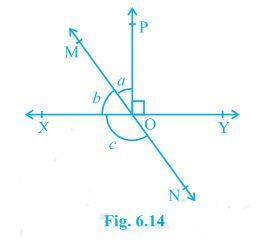# In figure lines XY and MN intersect at O. If ∠POY = 90° and a : b = 2 : 3, find c.### Solution

We know that the sum of linear pair is always equal to 180°

So,

∠POY +a +b = 180°

Putting the value of ∠POY = 90° (as given in the question) we get,

a+b = 90°

Now, it is given that a : b = 2 : 3 so,

Let a be 2x and b be 3x

∴ 2x+3x = 90°

Solving this we get

5x = 90°

So, x = 18°

∴ a = 2×18° = 36°

Similarly, b can be calculated and the value will be

b = 3×18° = 54°

From the diagram, b+c also forms a straight angle so,

b+c = 180°

c+54° = 180°

∴ c = 126°

Check out the video given below to know more about the types of anglesArticles to explore(1)(0)